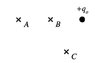# Electric potential question

cy19861126
Homework Statement
A positive charge of magnitude qo is shown in the diagram below. Points B and C are a distance r away from the charge and point A is a distance 2r from it.
1. Would the absolute value of work done by an external agent in moving the same test charge from point B to C be greater than, less than, or equal to from point A to B

2. Would the absolute value of the work done by an external agent in moving the same test charge from point A to C be greater than, less than, or equal to from point A to B?

W = Fd

## The Attempt at a Solution

1. Work from A to B to be would be negative because the distance is in the opposite direction of the force being exerted. From B to C would be 0 because the first half of the path has a negative work done and the second half of the path has a positive work being done. The absolute work done would be 0 because both paths have equal magnitude but different direction. Therefore, work from point B to C would be greater than from point A to B

2. I really have no idea about this problem. From A to C and from A to B are both negative, which makes the problem incomparable. All I know is that A to C has a longer distance than from A to B. However, I cannot compare their forces...

#### Attachments

•TUT 2a.jpg
3.7 KB · Views: 704
Last edited:

Staff Emeritus
Gold Member
1. Work from A to B to be would be negative because the distance is in the opposite direction of the force being exerted.

You don't know that. In order to be able to say that with certainty, you would have to have been told that there is a nonzero external electric field pointing from B to A. You weren't told anything like that.

Actually, I don't see how you can answer the question at all. If the field is zero, then it takes zero work to move the particle along any path (at constant velocity, that is). So let's assume that there is a constant, uniform external electric field. In that case then this problem becomes one of simple geometry. You know that $F=q_0E$ is a constant, so you only have to determine which distance is greater when finding the absolute value of the work. And do note that the fact that they ask about the absolute value of the work allows you to disregard the sign of $W$.

So to answer the questions all you have to do is decide which path is longer.

cy19861126
How can the electric field be constant? E = k*(q/r^2) As you can see, electric field is dependent on the distance on the point charge